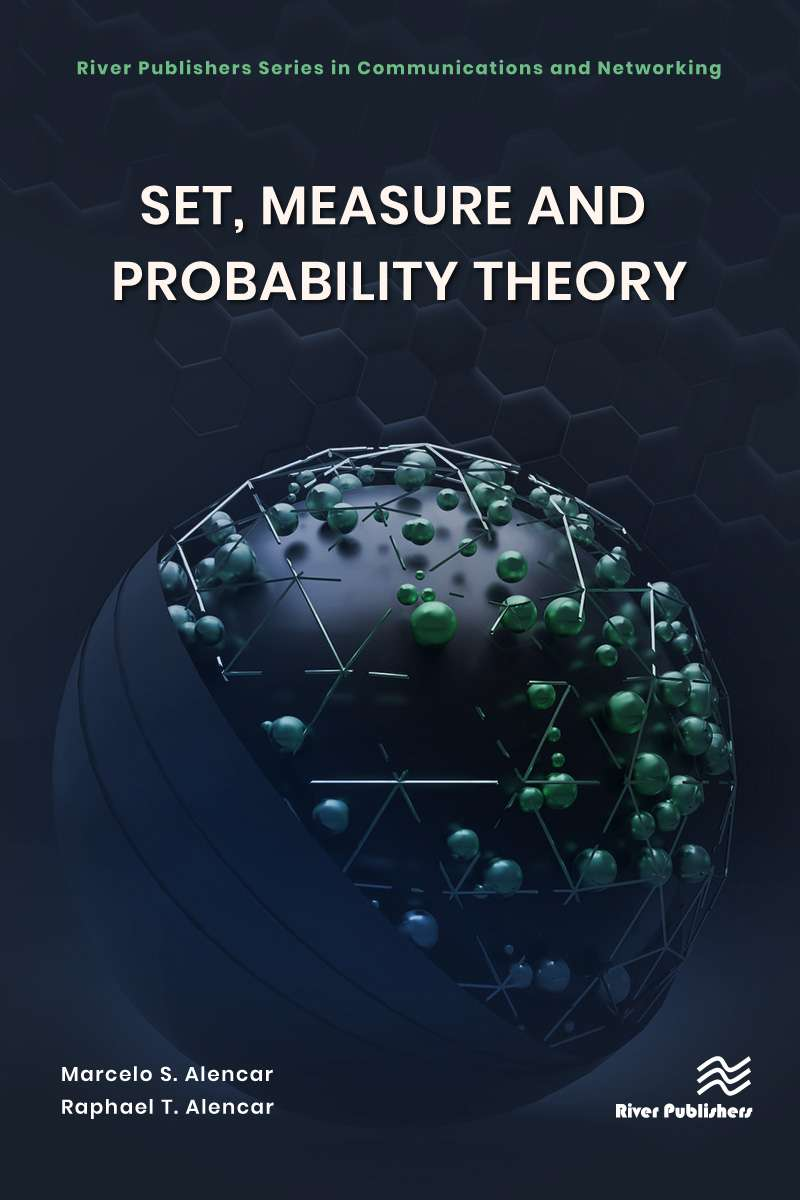Set, Measure, and Probability Theory

River Publishers Series in Mathematical, Statistical and Computational Modelling for Engineering

##### Set, Measure, and Probability Theory Forthcoming

Authors:
Marcelo S. Alencar, Institute for Advanced Studies in Communications (Iecom) Federal University of Rio Grande do Norte (UFRN), Brazil
Raphael T. Alencar, Raydiall Automotive, France

ISBN: 9788770228473 e-ISBN: 9788770228824

Available: October 2023

This book introduces the basic concepts of set theory, measure theory, the axiomatic theory of probability, random variables and multidimensional random variables, functions of random variables, convergence theorems, laws of large numbers, and fundamental inequalities. The idea is to present a seamless connection between the more abstract advanced set theory, the fundamental concepts from measure theory, and integration, to introduce the axiomatic theory of probability, filling in the gaps from previous books and leading to an interesting, robust and, hopefully, self-contained exposition of the theory.

This book also presents an account of the historical evolution of probability theory as a mathematical discipline. Each chapter presents a short biography of the important scientists who helped develop the subject. Appendices include Fourier transforms in one and two dimensions,  important formulas and inequalities and commented bibliography. Many examples, illustrations and graphics help the reader understand the theory.

Probability theory, measure theory, set theory.